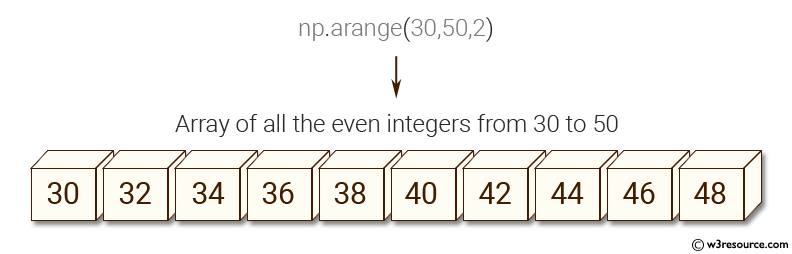﻿ NumPy: Create an array of all the even integers from 30 to 70 - w3resource

# NumPy: Create an array of all the even integers from 30 to 70

## NumPy: Basic Exercise-15 with Solution

Write a NumPy program to create an array of all the even integers from 30 to 70.

Sample Solution :

Python Code :

``````import numpy as np
array=np.arange(30,71,2)
print("Array of all the even integers from 30 to 70")
print(array)
``````

Sample Output:

```Array of all the even integers from 30 to 70
[30 32 34 36 38 40 42 44 46 48 50 52 54 56 58 60 62 64 66 68 70]
```

Pictorial Presentation:Python Code Editor:

Have another way to solve this solution? Contribute your code (and comments) through Disqus.

What is the difficulty level of this exercise?

﻿

Inviting useful, relevant, well-written and unique guest posts